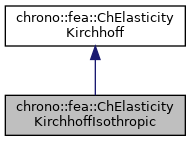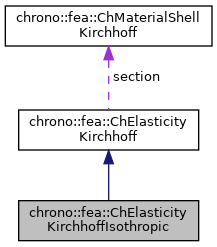chrono::fea::ChElasticityKirchhoffIsothropic Class Reference

## Description

Isothropic elasticity for thin shells (Kirchoff-Love shell theory, without shear effects) to be used in a ChMaterialShellKirchhoff.

This class implements material properties for a layer of a Kirchhoff thin shell, for the case of isotropic linear elastic material. This is probably the material that you need most often when using thin shells.

#include <ChMaterialShellKirchhoff.h>

Inheritance diagram for chrono::fea::ChElasticityKirchhoffIsothropic:[legend]
Collaboration diagram for chrono::fea::ChElasticityKirchhoffIsothropic:[legend]

## Public Member Functions

ChElasticityKirchhoffIsothropic (double E, double nu)
Construct an isotropic material. More...

double Get_E () const
Return the elasticity moduli.

double Get_nu () const
Return the Poisson ratio.

virtual void ComputeStress (ChVector<> &n, ChVector<> &m, const ChVector<> &eps, const ChVector<> &kur, const double z_inf, const double z_sup, const double angle) override
The FE code will evaluate this function to compute per-unit-length forces/torques given the strains/curvatures. More...

virtual void ComputeStiffnessMatrix (ChMatrixRef mC, const ChVector<> &eps, const ChVector<> &kur, const double z_inf, const double z_sup, const double angle) override
Compute 12x12 stiffness matrix [Km] , that is [ds/de], the tangent of the constitutive relation per-unit-length forces/torques vs generalized strains. More...Public Attributes inherited from chrono::fea::ChElasticityKirchhoff
ChMaterialShellKirchhoffsection

## ◆ ChElasticityKirchhoffIsothropic()

 chrono::fea::ChElasticityKirchhoffIsothropic::ChElasticityKirchhoffIsothropic ( double E, double nu )

Construct an isotropic material.

Parameters
 E Young's modulus nu Poisson ratio

## ◆ ComputeStiffnessMatrix()

 void chrono::fea::ChElasticityKirchhoffIsothropic::ComputeStiffnessMatrix ( ChMatrixRef mC, const ChVector<> & eps, const ChVector<> & kur, const double z_inf, const double z_sup, const double angle )
overridevirtual

Compute 12x12 stiffness matrix [Km] , that is [ds/de], the tangent of the constitutive relation per-unit-length forces/torques vs generalized strains.

Parameters
 mC tangent matrix eps strains e_11, e_22, e_12 kur curvature k_11, k_22, k_12 z_inf layer lower z value (along thickness coord) z_sup layer upper z value (along thickness coord) angle layer angle respect to x (if needed)

Reimplemented from chrono::fea::ChElasticityKirchhoff.

## ◆ ComputeStress()

 void chrono::fea::ChElasticityKirchhoffIsothropic::ComputeStress ( ChVector<> & n, ChVector<> & m, const ChVector<> & eps, const ChVector<> & kur, const double z_inf, const double z_sup, const double angle )
overridevirtual

The FE code will evaluate this function to compute per-unit-length forces/torques given the strains/curvatures.

Parameters
 n forces n_11, n_22, n_12 (per unit length) m torques m_11, m_22, m_12 (per unit length) eps strains e_11, e_22, e_12 kur curvature k_11, k_22, k_12 z_inf layer lower z value (along thickness coord) z_sup layer upper z value (along thickness coord) angle layer angle respect to x (if needed) -not used in this, isotropic

Implements chrono::fea::ChElasticityKirchhoff.

The documentation for this class was generated from the following files:
• /builds/uwsbel/chrono/src/chrono/fea/ChMaterialShellKirchhoff.h
• /builds/uwsbel/chrono/src/chrono/fea/ChMaterialShellKirchhoff.cpp